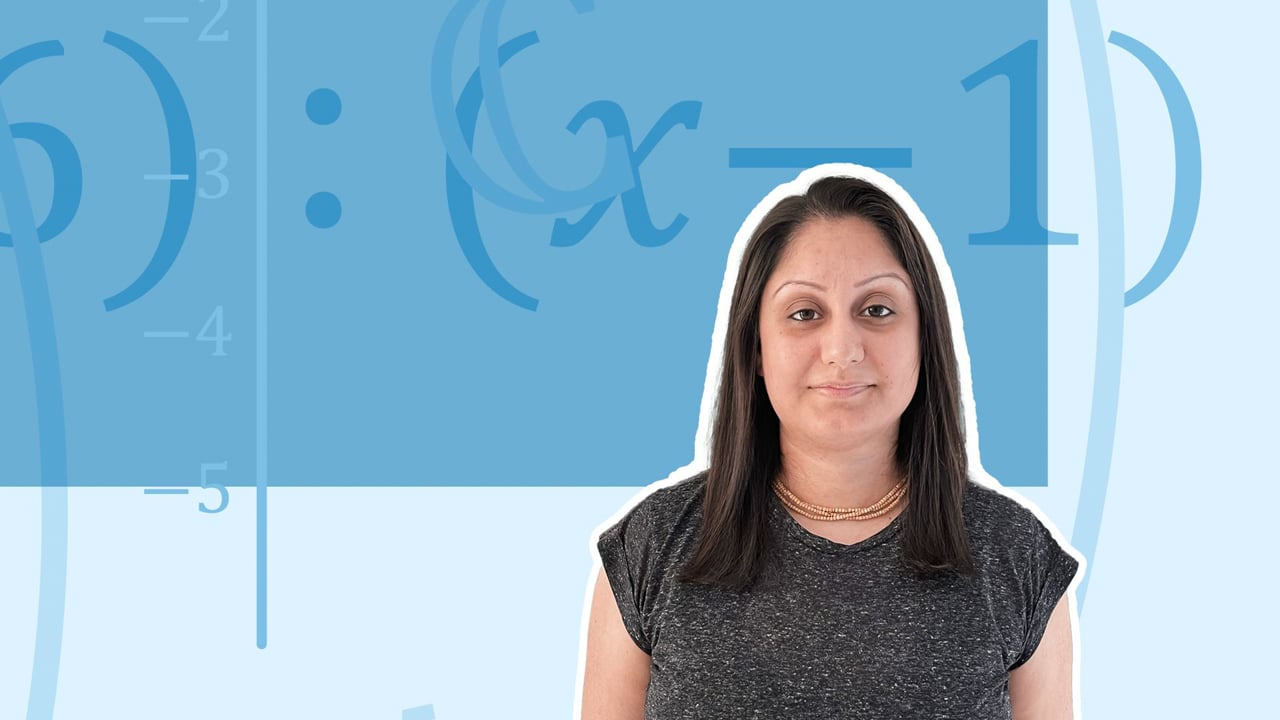Chapter OverviewMaths

Types of numbers

Number calculations

Fractions, decimals and percentages

Algebraic manipulation

Formulae and equations

Straight line graphs

Other graphs

Ratio

Proportion

Rates of change

Shapes

Properties of shapes

Measures

Lines and angles

Drawing shapes

Trigonometry

Probability

Statistics

Maths

# Substituting numbers into an expression0%

Summary

# Substituting numbers into an expression

## ​​In a nutshell

Substitution means replacing the variable with a number. Substitution helps find the value of simple expressions and formulae. You should be able to substitute positive or negative numbers. Take care when substituting in for more than one variable or with powers.

## Recap on negative numbers

 ​$+ \times + = +$​​ ​$+ \times - = -$​​ ​$- \times + = -$​​ ​$- \times - = +$​​

## Substitution

Substitute the value into the expression and then calculate the answer.

##### Example 1

Find the value of $6n+7$ when $n=8$.

\begin {aligned}6n+7 &= 6 \times 8 + 7 \\ &=\underline {55} \\\end {aligned}​​

##### Example 2

Find the value of $6n+7$ when $n=-2$.

\begin {aligned}6n+7 &= 6 \times -2 + 7 \\ &= \underline{-5} \\\end {aligned}​​

##### Example 3

Find the value of $\frac {ab} c$ when $a=15, b=4, c=3$.

\begin {aligned}\frac {ab} c &= \frac {15 \times 4} 3 \\ &= \underline{20} \\\end {aligned}​​

##### Example 4

The volume of the cuboid is given by $V = Lwh$. Find the volume when $L=5, w=2, h=3$.

\begin {aligned}V &= L \times w \times h \\& = 5 \times 2 \times 3 \\&= \underline{30} \end {aligned}​​

FAQs

• Question: What are the rule for multiplying negative numbers?

Answer: When multiplying two numbers, if one of the numbers is positive and one is negative, then the answer will be negative. If both numbers are negative, then the answer will be positive.

• Question: What is substitution?

Answer: Substitution means replacing. In algebra we replace the variable (or letter) with a number.

• Question: What is substituting into an expression?

Answer: Substitution means replacing the variables in an algebraic expression with their numerical values. We can then work out the value of the expression.

Theory

Exercises